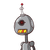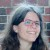# Factorise the following by taking out the common factor6xy – 4y2 + 12xy – 2yzx​

Factorise the following by taking out the common factor
6xy – 4y2 + 12xy – 2yzx​

### 2 thoughts on “Factorise the following by taking out the common factor<br />6xy – 4y2 + 12xy – 2yzx​”

1.## ✎Δnsɯer࿐

6xy + 12xy – 4y2 – 2yzx [∵ Addition is commutative]

= (6 × x × y) + (2 × 6 × x × y) + (–1)(2)(2) y + y) + ((–1)(2)(y)(z)(x))

Taking out 6 x x x y from first two terms and (–1) × 2 × y from last two terms we get

= 6 × x × y(1 + 2) + (–1)(2)y [2y + zx]

= 6 × y(3) – 2y(2y + zx)

= (2 × 3 × 3 × x × y) – 2xy(2y + zx)

Taking out 2y from two terms

= 2y(9x – (2y + zx))

= 2y(9x – 2y – xz)

2.6xy + 12xy – 4y2 – 2yzx [Addition is commutative]

= = (6 × x × y) + (2 × 6 × x × y) + (−1)(2)(2) y +

y) + ((-1)(2)(y)(z)(x))

Taking out 6 x x x y from first two terms and (-1) × 2 × y from last two terms we

get

= 6 x × x × y(1 + 2) + (−1)(2)y [2y+zx]

= 6 xy(3) = 2y(2y+zx)

= (2 × 3 ×3 × x × y) – 2xy(2y + zx)

Taking out 2y from two terms

= 2y(9x – (2y + zx))

= 2y(9x – 2y – xz)